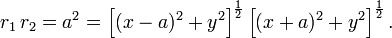# Lemniscate

.

A lemniscate is a geometric curve in the form of the digit 8, usually drawn such that the digit is lying on its side, as the infinity symbol$\infty$. The name derives from the Greek λημνισκος (lemniskos, woolen band).

Two forms are common.

## Lemniscate of Gerono

This form is named for the French mathematician Camille Christophe Gerono (1799-1891). Its equation in Cartesian coordinates is$\ x^4 = a^2 (x^2 - y^2)$.

The figure shows the case a = √2

## Lemniscate of Bernoulli

This form was discovered by James Bernoulli, who coined the term Curva Lemniscata, comparing the curve to a noeud de ruban (a ribbon knot) in an article in Acta Eruditorum of September 1694 (p. 336). Basically, Bernoulli's lemniscate is the locus of points that have a distance r1 to a focus F1 and a distance r2 to a focus F2, while the product r1×r2 is constant. In the figure the foci are on the x-axis at ±1. The product of the distances is constant and equal to half the distance 2a between the foci squared. For foci on the x-axis at ±a the equation is,$r_1\,r_2 = a^2 = \left[ (x-a)^2 + y^2\right]^{\frac{1}{2}} \left[(x+a)^2 + y^2\right]^{\frac{1}{2}}.$

Expanding and simplifying gives$(x^2 + y^2)^2 = 2a^2 (x^2 - y^2).\;$

The latter equation gives upon substitution of$x=r\cos\theta,\quad y = r\sin\theta$

the following polar equation$r^4 = 2 a^2 r^2 (\cos^2\theta - \sin^2\theta) \Longrightarrow r^2 = 2 a^2 \cos2\theta.$

Bernoulli's lemniscate belongs to the more general class of the Cassini ovals.